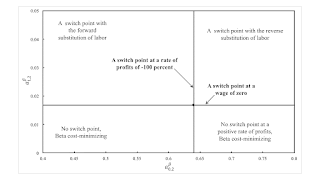Wednesday , February 1 2023Home / Post-Keynesian / The Emergence Of The Reverse Substitution Of Labor

# The Emergence Of The Reverse Substitution Of Laborby
Robert Vienneau
My articles My siteMy booksMy videos
Summary:
Figure 1: A Wage Frontier With Two Fluke Switch Points This post is a rewrite of this, without the attempt to draw a connection to structural economic dynamics. This post presents an example with circulating capital alone. Table 1 presents the technology for an economy in which two commodities, iron and corn, are produced. Managers of firms know of one process for producing iron and two for producing corn. Each process is specified by coefficients of production, that is, the required physical inputs per unit output. The Alpha technique consists of the iron-producing process and the first corn-producing process. Similarly, the Beta technique consists of the iron-producing process and the second corn-producing process. At any time, managers of firms face a problem of the choice of

Topics:
Robert Vienneau considers the following as important: , ,

This could be interesting, too:

Robert Vienneau writes CCC Does Not Depend On Reswitching

Robert Vienneau writes Reswitching Of The Order Of Fertility

Robert Vienneau writes Reswitching Of The Order Of Rentability

Robert Vienneau writes A Fluke Case For Extensive RentFigure 1: A Wage Frontier With Two Fluke Switch Points

This post is a rewrite of this, without the attempt to draw a connection to structural economic dynamics.

This post presents an example with circulating capital alone. Table 1 presents the technology for an economy in which two commodities, iron and corn, are produced. Managers of firms know of one process for producing iron and two for producing corn. Each process is specified by coefficients of production, that is, the required physical inputs per unit output. The Alpha technique consists of the iron-producing process and the first corn-producing process. Similarly, the Beta technique consists of the iron-producing process and the second corn-producing process. At any time, managers of firms face a problem of the choice of technique.

 Input Iron Industry Corn Industry Alpha Beta Labor a0,1 = 1 a0,2α = 16/25 a0,2β Iron a1,1 = 9/20 a1,2α = 1/625 a1,2β Corn a2,1 = 2 a2,2α = 12/25 a2,2β = 27/400

Two parameters are not given numerical values in this specification of technology. The approach taken here is to examine a local perturbation of parameters in a two-dimensional slice of the higher dimensional parameter space defined by the coefficients of production in particular numeric examples. With wages paid out of the surplus product at the end of the period of production, the wage curves for the two techniques are depicted in Figure 1 for a particular parametrization of the coefficients of production. The Beta technique is cost-minimizing for any feasible distribution of income. If the wage is zero and the workers live on air, the Alpha technique is also cost-minimizing.

A switch point is defined in this model of circulating capital to be an intersection of the wage curves. These switch points, for the particular parameter values illustrated in Figure 1, are fluke cases. Almost any variation in the model parameters destroys their interesting properties. A switch point exists at a rate of profits of -100 percent only along a knife edge in the parameter space (Figure 2). Likewise, a switch point exists on the axis for the rate of profits only along another knife edge. The illustrated example, with two fluke switch points, arises at a single point in the parameter space, where these two partitions intersect.Figure 2: The Parameter Space for the Reverse Substitution of Labor

Figure 2 depicts a partition of the parameter space around the point with these two fluke switch points. Below the horizontal line, the switch point on the axis for the rate of profits has disappeared below the axis. The Beta technique is cost-minimizing for all feasible non-negative rates of profits. Above this locus, the Alpha technique is cost-minimizing for a low enough wage or a high enough feasible rate of profits.

In the northwest, the switch point at a negative rate of profits occurs at a rate of profits lower than 100 percent. Around the switch point at a positive rate of profits, a lower wage is associated with the adoption of the corn-producing process with a larger coefficient for labor. That is, at a higher wage, employment is lower per unit of gross output in the corn industry.

In the northeast of Figure 2, the switch point for a positive rate of profits exhibits the reverse substitution of labor. Around this switch point, a higher wage is associated with the adoption of a process producing the consumer good in which more labor is employed per unit of gross output. The other switch point exists for a rate of profits between -100 percent and zero. Steedman (2006) presents examples with this phenomenon in models with other structures.

Qualitative changes in the wage frontier exist in the parameter space away from the part graphed in Figure 2. The analysis presented here is of local perturbations of the depicted fluke case.